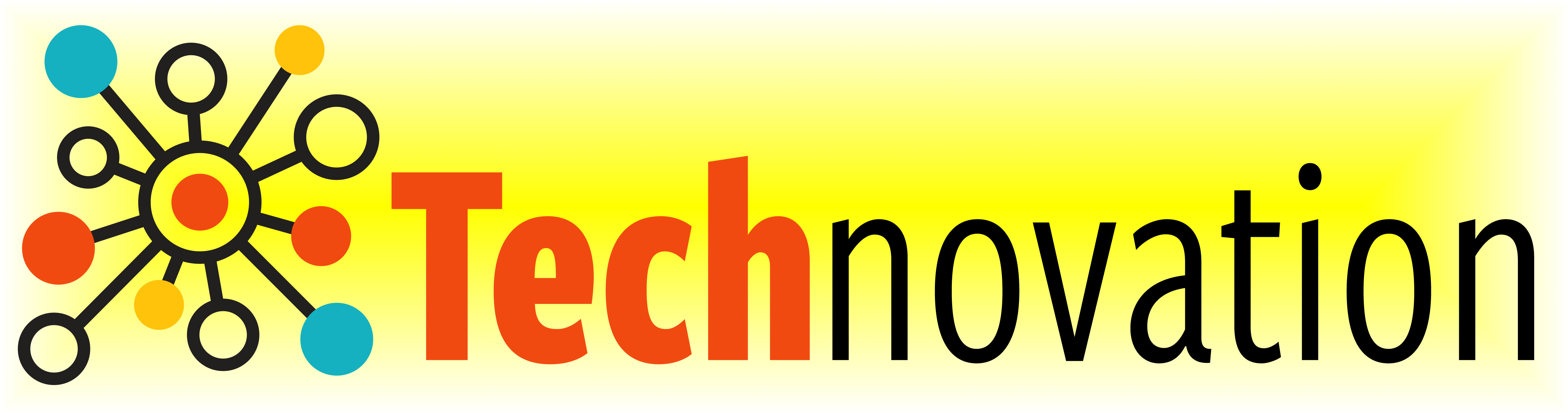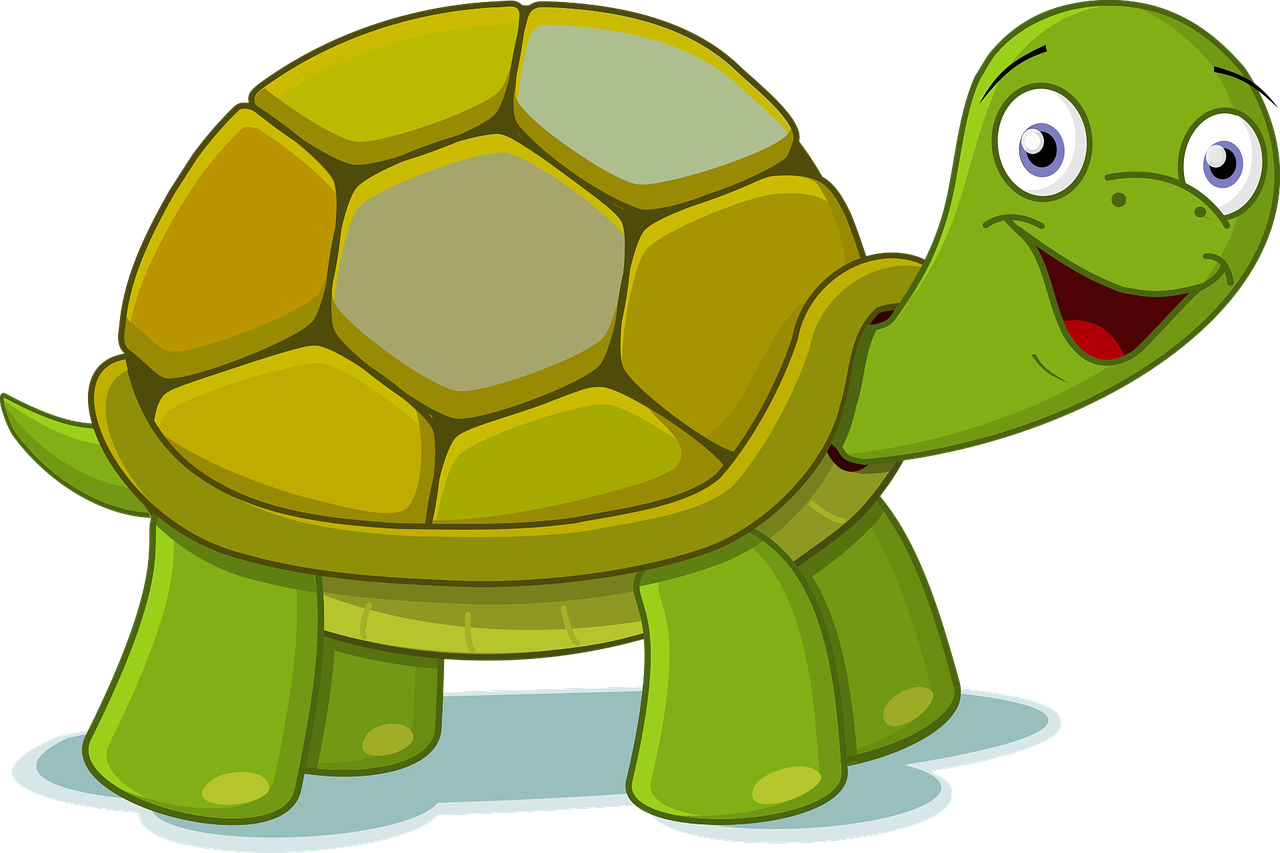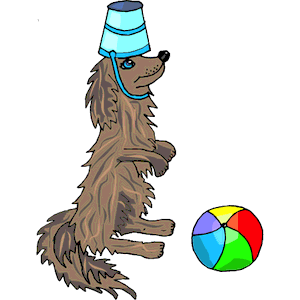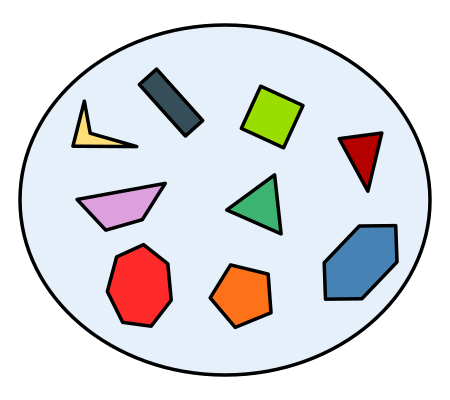# 2.4. Learning to Code¶

## 2.4.1. Python Turtle¶

Think of a Python `turtle` as your new (virtual) pet. Instead of using words and gestures to teach it to roll over or sit, you write computer programs containing instructions that tell it how to draw pictures on a screen.

For example, the code in the box below instructs the `turtle` to draw a square.

Press the `Run` button. You may need to scroll the window to see the screen below the editor window.

Check your understanding of this program.

Arrange the instructions into a program that draws a rectangle 150 pixels wide and 100 pixels high, like the Rectangle Image shown above.

You can instruct a (Python) `turtle` to do much more than just to move `forward` and turn `left`. Following are some other instructions that you can give it.

See if you can guess what each does. Then press the button to check if you guessed correctly.

With these commands, we can instruct the `turtle` to draw more interesting diagrams.

For example, here’s a program that draws a six-pointed star in two colors.

Run the program and scroll down to see what the `turtle` draws.

The computer executes the instructions in a program exactly as they are written and in the exact order. Your dog probably is not as obedient!Sometimes the order doesn’t matter; other times it does.

Arrange the instruction blocks below into a program that draws:

1. First, a purple circle of radius 75.

2. Then, a red circle of radius 50.

3. And finally, a blue circle of radius 25.

The drawing it produces should look like the Concentric Circles Image above.

(Drag the instruction blocks into the yellow rectangular region in the order that the computer should execute them.)

We’ll introduce more `turtle` instructions as we go along. But if you are curious, you can look here to learn all about Turtle Graphics, including all of the instructions that the `turtle` understands.

## 2.4.2. Python Basics¶

According to the:

The History of Programming Languages (HOPL) listed 8,512 different programming languages in January of 2011! No doubt, there are even more by now!

The animation below gives you an idea of how the popularity of modern programming languages has fluctuated in just the last 7 years. (With over 40 *million* users and hosting more than 190 *trillion* public code bases,hosts the largest collection of open-source software in the world.)

You might think that becoming an expert programmer is hopeless since there are so many languages—how could you hope to learn even a small fraction of them? But the good news is that you don’t need to. Almost all current languages allow you to do the same basic things. So just pick one of them. We chose Python for this ebook since it is relatively easy to learn compared to some others and is as powerful as any. Also, most programming languages are based on the same basic concepts.

The rest of this section introduces four such concepts: Keywords, types, variables, and assignment. We will need these concepts to create more interesting drawings in later meetings.

### 2.4.2.1. Keywords¶

All but the most primitive programming languages define words that mean something special to the computer. Called keywords, these words help the computer recognize the instructions that you want it to execute. There are 35 keywords in Python, as shown below.

Python Keywords

False

await

else

import

pass

None

break

except

in

raise

True

class

finally

is

return

and

continue

for

lambda

try

as

def

from

nonlocal

while

assert

del

global

not

with

async

elif

if

or

yield

Because these words already mean something to the computer, you can use them only in special instructions.

In later meetings, we’ll learn how to use many other keywords. For now, it’s enough to know that a keyword is a word that has a particular meaning in all programs.

### 2.4.2.2. Types¶

A type is a set of values that share some common properties and/or operators. Python has four built-in types; they are named: 1) `int`, 2) `float`, 3) `bool`, and 4) `str`.

The first two are both numeric types. An `int` represents an integer (or a whole number). The numeric values in our examples so far have all been `int`’s. In contrast, a `float` represents a decimal number. The computer figures out if a number is an `int` or a `float` by how you write it: a number with no decimal point is an `int` and one containing a decimal point is a `float`. For example, `2` and `-33` are `int`’s and `-2.0` and `1.414` are `float`’s. The numeric types share the usual arithmetic operators—addition (`+`), subtraction (`-`), multiplication (`*`), division (`/`), and so on—and also some more advanced operators, which we probably won’t need to use in this ebook.

The `bool` type has only two logical values: `True` and `False`. In later weeks, we’ll be using logical values and operators in instructions that require decision making. Remember that Python is case-sensitive: For example, `true` is not the same as `True`; and neither is `TRUE`.

The term `str` is short for string, which represents a series of characters (or text). You write a string by placing single quotes (`'`), double quotes (`"`), or triple double-quotes (`"""`) around its characters. The quotes that start and end a string have to match—in other words, if you start a string with a single quote, you have to end it with a single quote, and so on for the other quotes. For example,

`'Hello World!'`

`"Hello World!"` and

`"""Hello World!"""`

all represent the same 12-character string (the space and exclamation point are both characters).

### 2.4.2.3. Variables¶

If you did the practice exercises from our last meeting, you probably got the feeling that variables are useful. If so, you are right! Almost all programming languages allow coders to create variables.

A variable is like a container that you have labeled with a name (the variable name). When the computer executes a program, you can instruct it to store a value in the variable (container) and then use that value in a later instruction.

In Python, the name that you give to a variable has to satisfy three rules:

• It must consist of one or more lower case letters (`a` to `z`), upper case letters (`A` to `Z`), digits (`0` to `9`), and/or underscores (`_`).

• It cannot be identical to a Python keyword.

A good container label is one that reminds you what the container contains so you don’t have open it to find out. In much the same way, a good variable name is one that reminds you what your code will use the variable for. For example, you might name a variable `radius` if it will store the number of pixels in the radius of a circle or `time_left` if it will store the number of minutes left in a game.

### 2.4.2.4. Assignment¶

The instruction for storing a value in a variable is called an assignment. In Python, an assignment has the form

`var = exp`

where `var` is the name of a variable and `exp` is an expression. For example, `y = x + 1` is an assignment. The symbol `=` is called the assignment operator.

An expression is like a recipe that a computer can evaluate (execute) to create a value. Evaluation of the expression is said to return this value. The simplest expressions are values from the built-in types, like `5`, `True`, `-52.79`, or `"Name"`, and variables. Evaluation of a value just returns the value. Evaluation of a variable returns the value stored in the variable; so if `x` stores the value `5`, then evaluation of `x` returns `5`.

You can write more complex expressions by applying operators to expressions. For example, `x + 1` is an expression. When evaluated, it returns the value that is one more than the value stored in `x`. So if `x` stores `5`, evaluation of `x + 1` returns `6`.

We won’t list all the Python operators since you can easily look them up when you need them. But you can click on ‘Some Numeric Operators’ to see examples of the operators we will use in later examples. You should recognize most of these operators from your studies of arithmetic, although some may have been written differently.

An assignment instructs the computer to store the value returned by evaluating the expression on the right of the `=` in the variable on the left. For example, when the value of `x` is `5`, executing `y = x + 1` stores `6` (the value returned by evaluation `x + 1`) in `y`.

An experienced programmer will not read `y = x + 1` as: “y equals x plus one”Instead, they might read it as: “assign x plus one to y”

Or: “set y to x plus one”

Or: “y is assigned x plus one”

Or even: “y gets x plus one”

The code below is the beginning of a turtle graphics program that we’ll add to in the practice exercises for next week. See what you can understand of it by follow the instructions and calculating answers for the questions below.

```1
2  import turtle
3
4
5  ext_length = 100
6  ext_height = 150
7  border_width = 20
8  border_color = "tan"
9  inter_color = "green"
10
11
12 inter_length = ext_length - (2 * border_width)
13 inter_height = ext_height - (2 * border_width)
14
15
16 inter_area = inter_length * inter_height
17 border_area = (ext_length * ext_height) - inter_area
```